﻿

### On an autoregressive process driven by a sequence of Gaussian cylindrical random variables

#### Abstract

Let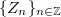$\{Z_n\}_{n\in\mathbb{Z}}$ be a sequence of identically distributed, weakly independent and weakly Gaussian cylindrical random variables in a separable Banach space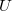$U$. We consider the cylindrical difference equation,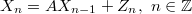$X_n=AX_{n-1}+Z_n,~{n\in\mathbb{Z}}$, in$U$ and determine a cylindrical process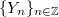$\{ Y_n\}_{n\in\mathbb{Z}}$ which solves the equation. The cylindrical distribution of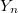$Y_n$ is shown to be weakly Gaussian and independent of$n$. It is also shown to be strongly Gaussian if the cylindrical distribution of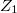$Z_1$ is strongly Gaussian. We determine the characteristic functional of$Y_n$ and give conditions under which$\{Y_n\}_{n\in\mathbb{Z}}$ is unique.

DOI Code: 10.1285/i15900932v41n1p111

Keywords: Autoregressive process; Cylindrical process; Cylindrical measure; Cylindrical random variable; Stationary process

Full Text: PDF